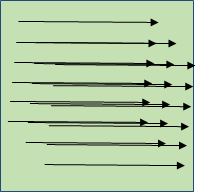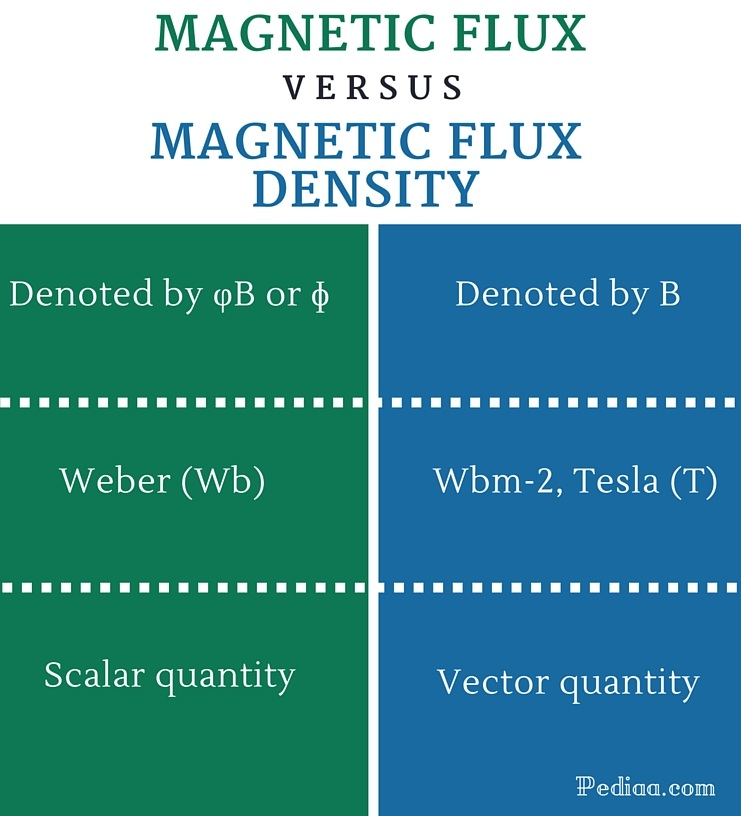# Difference Between Magnetic Flux and Magnetic Flux Density

## Main Difference – Magnetic Flux vs Magnetic Flux Density

In magnetism, several physical quantities such as magnetic flux, magnetic flux density and magnetic field strength are used to explain the behaviors or influences of magnetic fields. Some people use these terms interchangeably. But they have different and particular meanings. The main difference between magnetic flux and magnetic flux density is that magnetic flux is a scalar quantity whereas magnetic flux density is a vector quantity. Magnetic flux is the scalar product of the magnetic flux density and the area vector. This article tries to give clear explanations for magnetic flux and magnetic flux density.

## What is Magnetic Flux

Magnetic flux is a vital scalar quantity in magnetism. Usually, magnetic fields are visualized using magnetic field lines. The magnitude of a field is represented by the density of field lines. The arrows of the field lines represent the direction of the magnetic field. In terms of magnetic field lines, the magnetic flux through a given surface is directly proportional to the total number of field lines passing through it. However, the field lines are not real lines in space. They are just imaginary lines used as a simple model to explain the magnetic influences of moving charged particles and magnetic materials.

The magnetic flux in a constant magnetic field can be expressed mathematically as, ɸ = B.S.

ɸ is magnetic flux through the vector surface, B is the magnetic flux density and S is the area of the surface. In other words, the magnetic flux through a given surface area is equal to the scalar product (dot product) of the magnetic flux density and the area vector.

More generally, the magnetic flux can be expressed as ɸ = ∫∫ B.dS.

It can be easily shown that the magnetic flux through any closed surface is zero. But the magnetic flux through an open surface can be either zero or non-zero. An electromotive force is produced by a changing magnetic flux that passes through a conducting loop. This phenomenon is the basic working principle of generators. According to the Faraday’s law of induction, the magnitude of the electromotive force induced in a conducting loop by a changing magnetic flux is equal to the rate of change of the magnetic flux that linkages with the loop.## What is Magnetic Flux Density

The magnetic flux, which is also known as “magnetic induction” is another important quantity in magnetism. The magnetic flux density is defined as the amount of magnetic flux through a unit area placed perpendicular to the direction of magnetic field. It is a vector quantity, usually denoted by B.

The SI unit of magnetic flux density is Tesla (T). The Gauss (G) is the C.G.S unit of magnetic flux density; it is also commonly used, especially when dealing with weak magnetic flux densities because one Tesla is equal to 10000 G.

The magnetic flux density at a given point (δB), produced by a current element is given by the Biot- Savart equation. It can be expressed asHere, I is the current, δl is a vector with an infinitesimal magnitude, and rˆ is the unit vector of r. This is a very important equation when dealing with magnetic fields produced by current- carrying wires or circuits. The magnetic flux density produced by a current carrying wire depends on several factors such as the geometry of the wire, the magnitude and direction of the current and the position of the point at which the magnetic flux density is to be found. The Biot- Savart law is a combination of all those factors. So, it can be used to calculate the resultant magnetic flux density B, at any given point from a current- carrying wire.

The magnetic flux density (B) inside a material medium is equal to the magnetic permeability of that medium (µ) times the magnetic field strength (H). It can be expressed as B=µH. The magnetic permeability of ferromagnetic materials increases up to a particular value when the applied magnetic field strength increases. Thereafter, it decreases as the field strength increases further. So, the magnetic flux density also approaches a saturation level and then decreases when the magnetic field strength increases further, according to the equation B=µH. This phenomenon is known as the magnetic saturation.## Difference Between Magnetic Flux and Magnetic Flux Density

### Denoted by:

Magnetic flux: Magnetic flux is denoted by φB or ɸ.

Magnetic flux density: Magnetic flux density is denoted by B.

### SI units:

Magnetic flux: SI unit is Weber (Wb).

Magnetic flux density: SI units are Wbm-2, Tesla (T).

### Nature of the quantity:

Magnetic flux: Magnetic flux is a scalar.

Magnetic flux density: Magnetic flux density is a vector.